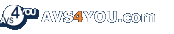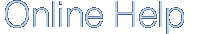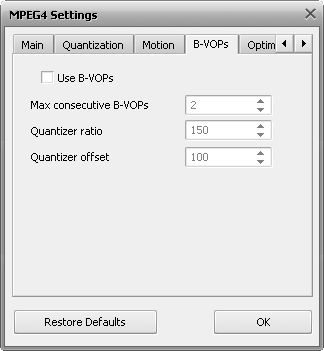Appendix
Appendix

# B-VOPs Settings[Use B-VOPs]   [Max consecutive B-VOPs]   [Quantizer ratio]   [Quantizer offset]

Use B-VOPs - an option that allows the codec algorithm to use so called bidirectional frames that are much smaller in size than usual frames and are predicted based on the frames before and after them. They let the codec increase the compression ratio without quality loss.

In video compression three types of frames can be used: I-, P- and B-frames. I-frames are the frames with a very small compression ratio. They are also called keyframes (intraframes) - see Max key interval. When watching the film the navigation is done using the I-frames. Between two I-frames the codec uses a succession of P-frames (delta frames or interframes), which have a much higher compression ratio, for instance:

 I P P P P P P P P P P P P P P P P I P P P P P P P

and so on. To further increase the video compression ratio, B-frames were introduced. They are inserted between two less compressed frames - I-frames or P-frames, so that the succession of the frames in the video file looks like

 I P B P B P B P B P B P B P B P I P B P B P B P B

Compression ratio for the B-frames is the highest among all the frames as they are compared with the previous and the following frames and only difference between them is written into B-frames. The released compression possibility is redistributed between the I-frames and P-frames allowing to compress them less but at the same time keeping the file size smaller. And though the quality of B-frames is worse than the quality of P-frames and I-frames, the picture quality remains quite good due to the reduction of compression of the latters.

It is recommended to leave the Use B-VOPs setting On.

Use B-VOPs setting can be either checked or unchecked.

Max consecutive B-VOPs - maximum number of sequential bidirectional frames in the video stream. The best result might be achieved when bidirectional frames and interframes are interleaved thus the Max consecutive B-VOPs number is equal to 1. If you set this number to 0 the result will be the same as to uncheck the Use B-VOPs setting. At higher values the movie picture might flicker but you might try to change the value to see the result for yourselves.

Quantizer ratio - the ratio used to calculate the bidirectional frames quantizer according to the following formula:

B-frames quantizer = [(Quantizer of previous P-frame + Quantizer of following P-frame) * (B-frame quantizer ratio)/200 + (B-frame offset/100)].

As can be seen, if you increase the default value the ratio of bidirectional frames compression will be also increased decreasing the ratio of compression of the other frames thus improving their quality. At the same time the quality of bidirectional frames will get worse so you should check the output result. If the default value is decreased the ratio of bidirectional frames compression will also decrease thus increasing the compression of the other frames and reducing their quality or increasing the size of the output video file which is not recommended.

Quantizer offset - the offset used to calculate the bidirectional frames quantizer. It is not recommended to change this value although you can try to change it to compare the results.

If you are not sure which settings to use you can press the Default button to discard the changes and restore default settings.

When ready press the OK button to accept the changes you have made.

•   File Format Settings
•   AVS Programs Codec Settings